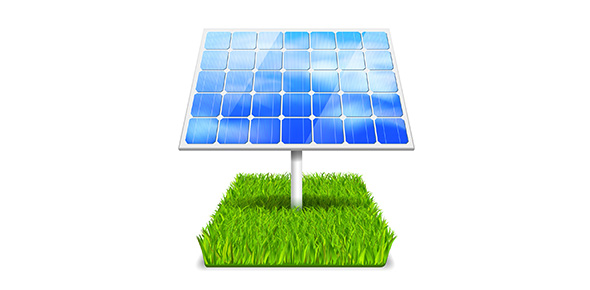# What Do You Know About Energy Transformations?

10 Questions | Total Attempts: 505SettingsCovers the following GPS Standards S8P2. Students will be familiar with the forms and transformations of energy. A. Explain energy transformation in terms of the Law of Conservation of Energy. B. Explain the relationship between potential and kinetic energy. C. Compare and contrast the different forms of energy (heat, light, electricity, mechanical motion, sound) and their characteristics.

• 1.
What two forms of energy combine to make mechanical energy?
• A.

Thermal and Electrical

• B.

Electrical and Kinetic

• C.

Potential and Thermal

• D.

Kinetic andPotential

• 2.
What type of energy is produced and wasted during most energy transformations?
• A.

Chemical

• B.

Thermal

• C.

Electrical

• 3.
You notice after you walk across a room, you feel a spark when you touch the doorknob. What energy transformation must have taken place?
• A.

Thermal --> electromagnetic

• B.

Mechanical --> electrical

• C.

Electromagnetic --> mechanical

• 4.
In what state is chemical energy always in?
• A.

Kinetic

• B.

Potential

• 5.
What energy transformation takes place when something burns?
• A.

Chemical --> thermal

• B.

Electrical --> mechanical

• C.

Electromagnetics --> chemical

• 6.
What energy tranformation happens in a toaster?
• A.

Mechanical --> electrical

• B.

Electrical --> thermal

• C.

Thermal --> chemical

• 7.
When a turbine rotates to produce electricity what energy transformation takes place?
• A.

Thermal --> mechanical

• B.

Electrical --> electromagnetic

• C.

Chemical --> electrical

• D.

Mechanical --> electrical

• 8.
Wind has energy because
• A.

It change direction.

• B.

It can do work.

• C.

It has mass.

• D.

It is electricaly charged.

• 9.
The ability to do work or cause change is called _________.
• A.

Energy

• B.

Transformation

• C.

Generator

• 10.
When you stretch a rubber band, you give it _______.
• A.

Kinetic enrgy.

• B.

Elastic potenital energy.

• C.

Gravitational potential energy.

• D.

Electrical energy.

Related TopicsBack to top